### Home > GC > Chapter 1 > Lesson 1.1.4 > Problem1-36

1-36.

The slope of a line is a measure of its steepness and indicates whether it goes up or down from left to right. For example, the slope of the line segment $A$ at right is $\frac{1}{2}$, while the slope of the line segment $B$ is $-\frac{3}{4}$.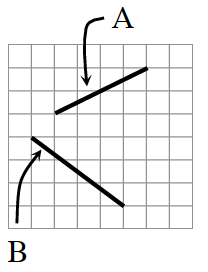For each line segment below, find the slope. You may want to copy each line segment on graph paper in order to draw slope triangles.

Create a slope triangle for each segment.

Find the change in $y$ and the change in $x$ for each segment.
See the example below. Note: $Δ =$ change.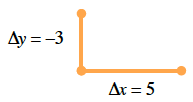1.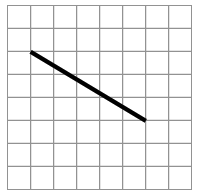$\frac{\Delta y}{\Delta x}=-\frac{3}{5}$

1.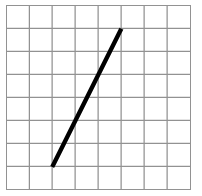1.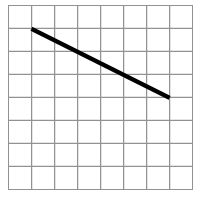1.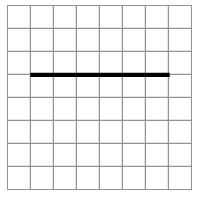$\frac{\Delta y}{\Delta x}=-\frac{0}{7}=0$